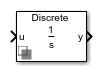# Integrator with Wrapped State (Discrete or Continuous)

Discrete-time or continuous-time integrator with wrapped state

• Library:
• Simscape / Electrical / Control / General Control

•## Description

The Integrator with Wrapped State (Discrete or Continuous) block implements a wrapped state integrator in conformance with IEEE 421.5-2016.

Use this block to generate periodic signals such as angles or to represent a voltage-controlled oscillator. You can switch between continuous and discrete implementations of the integrator using the Sample time parameter.

### Equations

Continuous

To configure the integrator for continuous time, set the Sample time property to `0`. This representation is equivalent to the continuous transfer function:

`$G\left(s\right)=\frac{1}{s}.$`

From the preceeding transfer function, the integrator defining equations are:

`$\left\{\begin{array}{c}\stackrel{˙}{x}\left(t\right)=u\left(t\right)\\ y\left(t\right)=x\left(t\right)\end{array}\text{\hspace{0.17em}}\text{\hspace{0.17em}}x\left(0\right)={x}_{0},$`

where:

• u is the integrator input.

• x is the integrator state.

• y is the integrator output.

• t is the simulation time.

• x0 is the initial state of the integrator.

Discrete

To configure the integrator for discrete time, set the Sample time property to a positive, nonzero value, or to `-1` to inherit the sample time from an upstream block. The discrete representation is equivalent to the transfer function:

`$G\left(z\right)=\frac{{T}_{s}}{z-1},$`

where Ts is the sample time. From the discrete transfer function, the integrator equations are defined using the forward Euler method:

`$\left\{\begin{array}{c}x\left(n+1\right)=x\left(n\right)+{T}_{s}u\left(n\right)\\ y\left(n\right)=x\left(n\right)\end{array}\text{\hspace{0.17em}}\text{\hspace{0.17em}}x\left(0\right)={x}_{0},$`

where:

• u is the integrator input.

• x is the integrator state.

• y is the integrator output.

• n is the simulation time step.

• x0 is the initial state of the integrator.

### Defining Initial Conditions

You can define the state initial conditions using Initial condition parameter.

### Wrapping Cyclic States

The integrator wraps its state between the specified lower and upper values. This diagram shows the outputs of a wrapped and nonwrapped state integrator for a constant input.In the diagram, the lower and upper limits are 0 and , respectively.

## Ports

### Input

expand all

Integrator input.

Data Types: `single` | `double`

### Output

expand all

Integrator output.

Data Types: `single` | `double`

## Parameters

expand all

Integrator upper limit.

Integrator lower limit.

Integrator initial state.

Time between consecutive block executions. During execution, the block produces outputs and, if appropriate, updates its internal state. For more information, see What Is Sample Time? and Specify Sample Time.

For inherited discrete-time operation, specify `-1`. For discrete-time operation, specify a positive integer. For continuous-time operation, specify `0`.

If this block is in a masked subsystem, or other variant subsystem that allows you to switch between continuous operation and discrete operation, promote the sample time parameter. Promoting the sample time parameter ensures correct switching between the continuous and discrete implementations of the block. For more information, see Promote Block Parameters on a Mask.

 IEEE Recommended Practice for Excitation System Models for Power System Stability Studies. IEEE Std 421.5-2016. Piscataway, NJ: IEEE-SA, 2016.